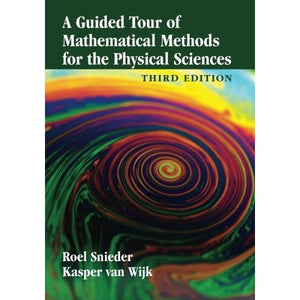># A Guided Tour of Mathematical Methods for the Physical Sciences

• £20.39
• Save £19

Roel Snieder
Cambridge University Press
Edition: 3, 3/5/2015
EAN 9781107641600, ISBN10: 1107641608

Paperback, 584 pages, 25.3 x 17.7 x 2.5 cm
Language: English

Mathematical methods are essential tools for all physical scientists. This book provides a comprehensive tour of the mathematical knowledge and techniques that are needed by students across the physical sciences. In contrast to more traditional textbooks, all the material is presented in the form of exercises. Within these exercises, basic mathematical theory and its applications in the physical sciences are well integrated. In this way, the mathematical insights that readers acquire are driven by their physical-science insight. This third edition has been completely revised: new material has been added to most chapters, and two completely new chapters on probability and statistics and on inverse problems have been added. This guided tour of mathematical techniques is instructive, applied, and fun. This book is targeted for all students of the physical sciences. It can serve as a stand-alone text, or as a source of exercises and examples to complement other textbooks.

1. Introduction
2. Dimensional analysis
3. Power series
4. Spherical and cylindrical coordinates
6. Divergence of a vector field
7. Curl of a vector field
8. Theorem of Gauss
9. Theorem of Stokes
10. The Laplacian
11. Scale analysis
12. Linear algebra
13. Dirac delta function
14. Fourier analysis
15. Analytic functions
16. Complex integration
17. Green's functions
principles
18. Green's functions
examples
19. Normal modes
20. Potential-field theory
21. Probability and statistics
22. Inverse problems
23. Perturbation theory
24. Asymptotic evaluation of integrals
25. Conservation laws
26. Cartesian tensors
27. Variational calculus
28. Epilogue on power and knowledge.